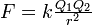# Point charges and their movement in 3 space

• Ic3Blu3
This magnetic field exerts a force on the other charge which adds to the electrostatic force. In summary, the question is asking for the ratio of the magnetic and electrostatic force on two equal charges when they are moving with a velocity. The electrostatic force is given by the equation kq^2/r^2, where k is the Coulomb's constant, q is the charge and r is the distance between the charges. The magnetic force is completely different and is given by the equation F = qvB, where q is the charge, v is the velocity and B is the magnetic field. The ratio of the magnitudes of these two forces can be found by dividing the magnetic force by the electrostatic force.

#### Ic3Blu3

Hey all this question appeared on my last assignment that was due on friday and had me completely stumped for the entire week and well still does, i couldn't even get off the ground (im not very good with these algebra questions) . anyway in the interests to doing my very best on my midterm exam I've come here in search of help.

## Homework Statement

Two equal charges q located at (0,0,0) and (0,b,0) at time zero are moving with speed v in the positive direction (v<<c). Find the ratio of the magnitudes of the magnetic and electrostatic force on each.

any help with this question would be much appreciated a starting point or a walk through of how to obtain the final result because i can't find anything on this in tipler. thankyou

i think I've made some progress but i know that the magnitude of the electrostatic force isbut how is this equation altered when a velocity is applied to it ?

well for the purposes of this question, the velocity since it is the same does not enter the equation you have posted.

Magnetic force completely different. Particles at rest, no magnetic interaction. so can you post an eqn or two that is concerned with magnetic fields, forces.

When the charges are at rest, the only force between them is the electrostatic force. But when the charges move with a velocity then a magnetic field is produced around them.